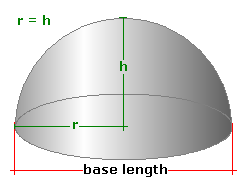Spike's Calculators

# Hemisphere Dome Tank Volume and Surface Area - MetresCalculate the volume of a dome-shaped tank that is a hemisphere (half sphere). Half of the length's base equals the height in this type of tank. This calculator uses metres for measurements.

##### Approximate weight of Fuel or Water
```one litre of water = 1 kilogram
one litre of diesel fuel = 0.832 kilogram
one litre of gas = 0.75 kilogram
```

### Hemispherical Dome Tank - Metres

Tank Diameter m
Liquid Weight-Kilograms per Litre kg/L

#### Results:

###### Tank Volume
In Cubic Metres
In Cubic Centimetres cm³
In Cubic Inches in³
In Cubic Feet ft³
###### Tank Capacity
In Litres L
In Hectolitres hl
In US Gallons gal US
In Imperial Gallons gal Imperial
###### Liquid Weight and Surface Area
Weight of Liquid kg
Surface Area in Square Metres
Surface Area in Square Centimetres cm²
Surface Area in Square Inches in²
Surface Area in Square Feet ft²

#### Calculator

1. enter the tank's base length in metres
2. the weight of the liquid in kilograms per litre

#### Results

1. the volume of the tank in cubic metres
2. the volume in cubic centimetres
3. the volume in cubic inches
4. the volume in cubic feet
5. the capacity of the tank in litres
6. the capacity in hectolitres
7. the capacity in US gallons
8. the capacity in Imperial gallons
9. full tank liquid weight based on kilograms/L
10. the surface area of the tank in square metres
11. the surface area in square centimetres
12. the surface area in square inches
13. the surface area in square feet

##### Formula
```V = 2/3Πr³
where V is the volume
Π = 3.14159265
r half the length of the tank base
```
##### Conversions
```one cubic metre (m³) = 1000000 cubic centimetres (cm³)
one cubic metre (m³) = 61023.7440947323 cubic inches (in³)
one cubic metre (m³) = 35.3146667214886 cubic feet (ft³)
one cubic metre (m³) = 1000 litres (L)
one cubic metre (m³) = 10 hectolitres (hl)
one cubic metre (m³) = 264.172052358148 gallons (gal) US
one cubic metre (m³) = 219.969248299088 gallons (gal) Imperial
one square metre (m²) = 10000 square centimetres (cm²)
one square metre (m²) = 1550.0031000062 square inches (in²)
one square metre (m²) = 10.7639104167097 square feet (ft²)
```

### Hemispherical Shaped Dome Tank/Container (Half Sphere)#### Filled